# Density and Specific Gravity - Practice Problems

Jump to: Rock and Mineral density | Rock and mineral specific gravity
You can download the questions (Acrobat (PDF) 25kB Jul24 09) if you would like to work them on a separate sheet of paper.

## Calculating densities of rocks and minerals

Problem 1: You have a rock with a volume of 15cm3 and a mass of 45 g. What is its density?

Problem 2: You have a different rock with a volume of 30cm3 and a mass of 60g. What is its density?

Problem 3: In the above two examples which rock is heavier? Which is lighter?

The question is asking about heavier and lighter, which refers to mass or weight. Therefore, all you care about is the mass in grams and so the 60 g rock in the second problem is heavierand the 45 g rock (in the first question) is lighter.

Problem 4: In the above two examples which rock is more dense? which is less dense?

The question is asking about density, and that is the ratio of mass to volume. Therefore, the first rock is denser, (density = 3.0) and the second rock is less dense even though it weighs more, because its density is only 2.0. This example shows why it is important to be careful to not use the words heavier/lighter when you means more or less dense.

Problem 5: You decide you want to carry a boulder home from the beach. It is 30 centimeters on each side, and so has a volume of 27,000 cm3. It is made of granite, which has a typical density of 2.8 g/cm3. How much will this boulder weigh?

In this case, you are asked for a mass, not the density. You will need to rearrange the density equation so that you get mass.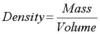By multiplying both sides by volume, mass will be left alone.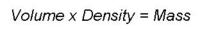Substituting in the values from the problem,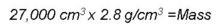The result is that the mass is 75,600 grams. That is over 165 pounds!

Problem 6: Rocks are sometimes used along coasts to prevent erosion. If a rock needs to weigh 2,000 kilograms (about 2 tons) in order not to be shifted by waves, how big (what volume) does it need to be? You are using basalt, which has a typical density of 3200 kg/m3
In this problem you need a volume, so you will need to rearrange the density equation to get volume.By multiplying both sides by volume, we can get volume out of the numerator (the bottom).You can then divide both sides by density to get volume alone: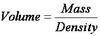By substituting in the values listed above,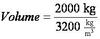So the volume will be 0.625 m3
Note that the above problem shows that densities can be in units other than grams and cubic centimeters. To avoid the potential problems of different units, many geologists use specific gravity (SG), explored in problems 8 and 9, below.

Problem 7: A golden-colored cube is handed to you. The person wants you to buy it for \$100, saying that is a gold nugget. You pull out your old geology text and look up gold in the mineral table, and read that its density is 19.3 g/cm3. You measure the cube and find that it is 2 cm on each side, and weighs 40 g. What is its density? Is it gold? Should you buy it?
To determine the density you need the volume and the mass since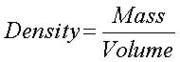.
You know the mass (40 g), but the volume is not given. To find the volume, use the formula for the volume of a box

volume = length x width x height.

The volume of the cube is
2cm x 2cm x 2cm = 8cm3.

The density then is the mass divided by the volume: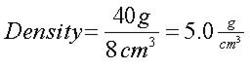Thus the cube is NOT gold, since the density (5.0 g/cm3) is not the same as gold (19.3g/cm3). You tell the seller to take a hike. You might even notice that the density of pyrite (a.k.a. fool's gold) is 5.0 g/cm3. Luckily you are no fool and know about density!

## Calculating Specific Gravity of Rocks and Minerals

Problem 8: You have a sample of granite with density 2.8 g/cm3. The density of water is 1.0 g/cm3. What is the specific gravity of your granite?

Specific gravity is the density of the substance divided by the density of water, so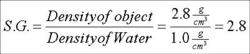Note that the units cancel, so this answer has no units. We say "the number is unitless."

Problem 9: You have a sample of granite with density 174.8 lbs/ft3. The density of water is 62.4 lbs/ft3. What is the specific gravity of the granite now?

Again, the specific gravity is the density of the substance divided by the density of water, so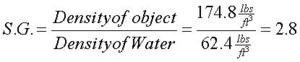This shows that the specific gravity does not change when measurements are made in different units, so long as the density of the object and the density of water are in the same units.

I think I've mastered density. Let me try the quiz
If this is not how you feel, you can go back to the explanations.